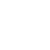Classes (extension) |

# FFTPower : UGen : AbstractFunction : ObjectExtension

Instantaneous spectral power

## Description

Outputs a power measurement (sum of squared magnitudes) taken from an FFT chain. It can be used for similar purposes as the Amplitude UGen but has different characteristics since it operates on the frequency-domain rather than time-domain representation.

The output is scaled by dividing by a factor related to the number of bins, meaning that output values occupy a similar dynamic range irrespective of the FFT frame size. To scale the power (e.g.) to lie between 0 and 1, you'll need to find an empirical value that suits the application - note the multipliers used in the examples below.

Note: The first implementation of this UGen summed the magnitudes without squaring them, which is not the ordinary definition of spectral power (it will behave in similar ways for some applications, but will have different characteristics e.g. the effect of noise). By default the UGen does now square the magnitudes, but you can achieve the old behaviour by setting the second "square" argument to false. For most uses you are recommended to leave this at its default.

## Class Methods

### FFTPower.kr(buffer, square: true)

#### Arguments:

 buffer square